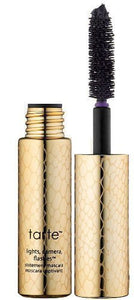# Tarte Lights, Camera, Flashes! Statement Mascara 5 Ml / 0.16 Fl Oz

• \$30.68
• Save \$1.18

• T

• a

• r

• t

• e

• L

• i

• g

• h

• t

• s

• ,

• C

• a

• m

• e

• r

• a

• ,

• F

• l

• a

• s

• h

• e

• s

• S

• t

• a

• t

• e

• m

• e

• n

• t

• M

• a

• s

• c

• a

• r

• a

• ,

• T

• r

• a

• v

• e

• l

• S

• i

• z

• e

• ,

• .

• 1

• 6

• O

• z

• .

• A

• l

• e

• n

• g

• t

• h

• e

• n

• i

• n

• g

• m

• a

• s

• c

• a

• r

• a

• t

• o

• i

• n

• t

• e

• n

• s

• i

• f

• y

• v

• o

• l

• u

• m

• e

• a

• n

• d

• c

• o

• n

• d

• i

• t

• i

• o

• n

• l

• a

• s

• h

• e

• s

• .

• T

• a

• r

• t

• e

• C

• o

• s

• m

• e

• t

• i

• c

• s

• L

• i

• g

• h

• t

• s

• C

• a

• m

• e

• r

• a

• F

• l

• a

• s

• h

• e

• s

• S

• t

• a

• t

• e

• m

• e

• n

• t

• M

• a

• s

• c

• a

• r

• a

• a

• d

• d

• s

• v

• o

• l

• u

• m

• e

• ,

• l

• e

• n

• g

• t

• h

• a

• n

• d

• d

• r

• a

• m

• a

• t

• o

• g

• i

• v

• e

• y

• o

• u

• r

• e

• y

• e

• s

• a

• d

• r

• a

• m

• a

• t

• i

• c

• e

• f

• f

• e

• c

• t

• .

• F

• o

• r

• m

• u

• l

• a

• t

• e

• d

• w

• i

• t

• h

• P

• r

• o

• v

• i

• t

• a

• m

• i

• n

• B

• 5

• ,

• i

• t

• n

• o

• u

• r

• i

• s

• h

• e

• s

• a

• n

• d

• e

• n

• s

• u

• r

• e

• s

• l

• a

• s

• h

• e

• s

• a

• r

• e

• s

• o

• f

• t

• a

• n

• d

• s

• h

• i

• n

• y

• .

• A

• n

• i

• n

• n

• o

• v

• a

• t

• i

• v

• e

• b

• r

• u

• s

• h

• w

• i

• t

• h

• s

• o

• f

• t

• b

• r

• i

• s

• t

• l

• e

• s

• c

• o

• m

• b

• s

• e

• a

• c

• h

• l

• a

• s

• h

• a

• n

• d

• p

• r

• e

• v

• e

• n

• t

• s

• m

• a

• s

• c

• a

• r

• a

• f

• r

• o

• m

• c

• l

• u

• m

• p

• i

• n

• g

• .

• Color : Black

Tarte Lights, Camera, Flashes Statement Mascara, Travel Size, .16 Oz. A lengthening mascara to intensify volume and condition lashes. Tarte Cosmetics Lights Camera Flashes Statement Mascara adds volume, length and drama to give your eyes a dramatic effect. Formulated with Provitamin B5, it nourishes and ensures lashes are soft and shiny. An innovative brush with soft bristles combs each lash and prevents mascara from clumping.

every pages